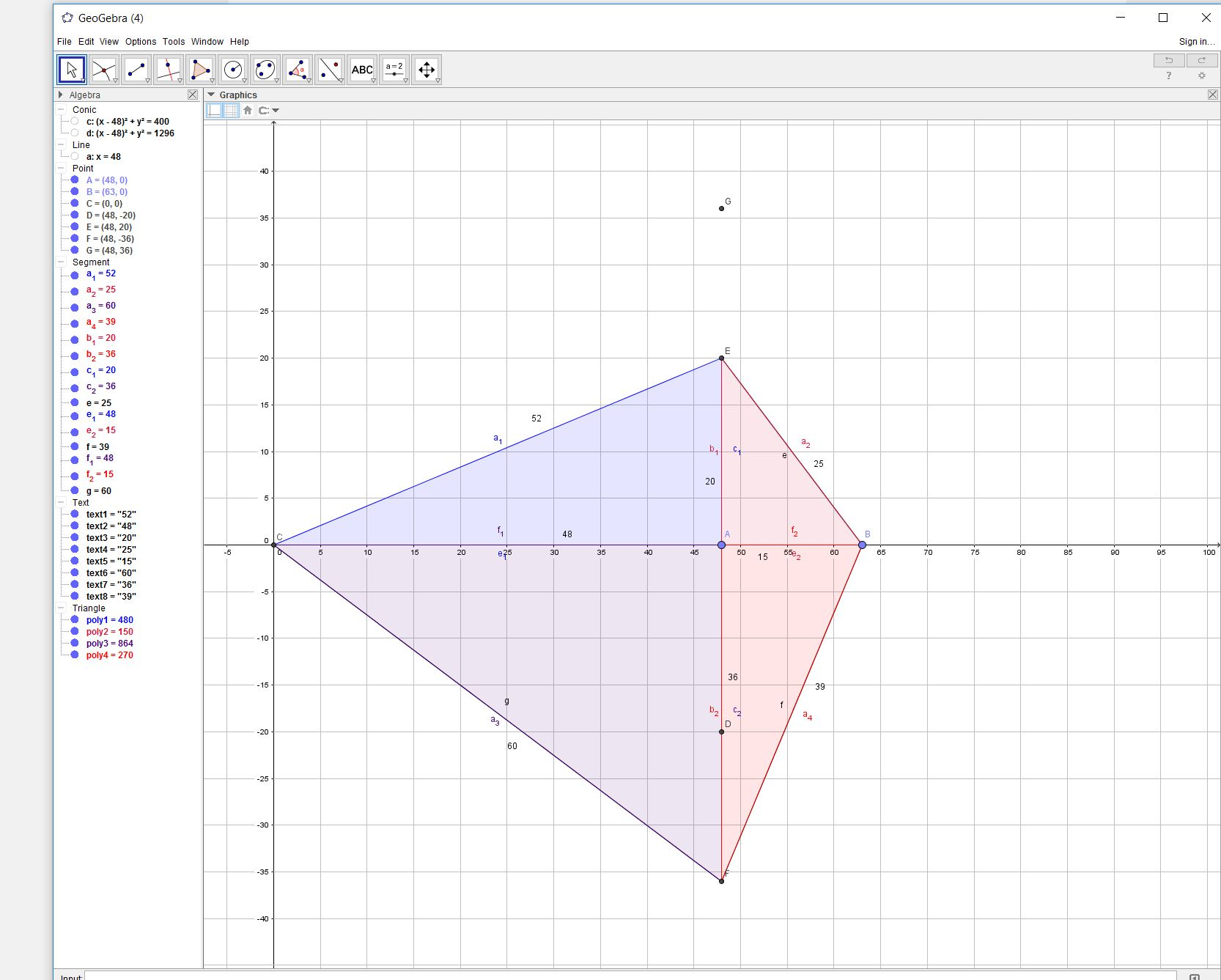Find a convex quadrilateral such that the quadrilateral has the smallest area and all four of its sides (a, b, c, d),   two diagonals (e, f) and area K are :

(1)   distinct integers
(2)   distinct prime integers

In a convex quadrilateral, the two diagonals cut the interior into four triangles, each with integer area.

What do you notice about the product of these areas?math grad - Interest: Number theory
This entry was posted in Number Puzzles and tagged . Bookmark the permalink.

### 7 Responses to Puzzle | convex quadrilateral

1.paul says:

This might be the one for part a

{a, b, c, d} = {52,25,39,60}
{e, f} = {63, 56}
area = 1764 sqr units

Here is a link to a diagramPaul

•paul says:

The product of areas is 360^4

P.

•benvitalis says:

(2) The area is always divisible by 6. so it is not possible to find a quadrilateral with a prime area

•benvitalis says:

(1) The area of triangle (10,17,21) is 84
The area of triangle (21,28,35) is 294
So the area of quadrilateral (10,17,28,35) is
84 + 294 = 378

2.paul says:

but one of the diagonals isn’t integer, its 36.5 in that arrangement.:)
P.

•benvitalis says:

This is what I get:
(10,17,21) –> 84
(21,28,35) –> 294
(10,35,39) –> 168
(17,28,39) –> 210

sides (10,17,28,35)
diagonals (21,39)

•paul says:

Yes you are right, I drew the opposite orientation of one of the triangles.